Latest Banking jobs   »

# Quantitative Aptitude Quiz For IBPS Clerk Prelims 2022- 18th July

Q1. A shopkeeper gains 20% profit but if he marks up the article 40% above cost price and gives a discount of 15%, he would get Rs. 30 less than before. Find the cost price of article?
(a) Rs. 4000
(b) Rs. 1000
(c) Rs. 3000
(d) Rs. 2000
(e) Rs. 5000

Q2. A started a business with investment of Rs. 30,000, after few months B joined the business with capital Rs. 40,000. If annual profit of A and B is Rs. 2700 and Rs. 2400 respectively. Then find after how many months B had joined the business?
(a) 6 months
(b) 4 months
(c) 8 months
(d) 2 months
(e) 10 months

Q3. In a partnership Pallavi invested Rs.2x for first four month and Rs.3x for next six months and Deepak invested Rs.4000 for 12 months. If Pallavi and Deepak got profit share in the ratio of 13 : 16 then, find the value of ‘8x-500’ ?
(a) 11500
(b) 21600
(c) 12400
(d) 23600
(e) 14000

Q4. Ayush buys an article for Rs. 800 and sold it at 20% profit and get some money and from that money he again buys the same article at previous cost but this time he sold it at 15% loss. Find his overall profit/loss percentage?
(a) 8%
(b) 12%
(c) 2%
(d) 5%
(e) 10%

Q5. A seller buys equal quantity of onion and tomato at a total of Rs. 20. If he sells onion at a profit of 25% and tomato at a loss of 15%, then find the S.P. of onion, if in whole transaction there is neither profit nor loss?
(a) 15.055 Rs.
(b) 12.975Rs.
(c) 6.375Rs.
(d) 9.375Rs.
(e) 11.665Rs.

Q6. An item marked at Rs.5000 was sold for Rs.3000 but later found there was mistake in giving discount, so discount was reduced by 10%. If cost price of same item was Rs.2960. Find the actual profit/loss? (in Rs)
(a)620
(b)240
(c)360
(d)540
(e)480

Q7. P, Q and R entered into a partnership by investing Rs.4800, Rs.3600 and Rs.5600 respectively. If at the end of a year profit earned by R is Rs.1260 then find the difference between the profit earned by P and Q? (in Rs.)
(a)720
(b)630
(c)540
(d)810
(e)270

Q8. A shopkeeper sold an article at 33⅓% profit and another at 16 ⅔% loss. Find the overall profit or loss percentage if cost price of both the articles is same?
(a)5%
(b)10%
(c) 8⅓%
(d)12.5%
(e)20%

Q9. Find the simple interest on Rs. X after 3 years at the rate of 12.5% p.a., if Rs. X is the compound interest earned on Rs.4800 in 2 years at the rate of 10% p.a.? (in Rs.)
(a)480
(b)448
(c)346
(d)422
(e)378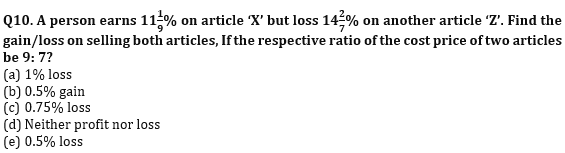Q11. P and Q enters into a partnership with Rs.1800 and Rs.2400 respectively. Find the total profit if profit share of P is Rs. 210 at the end of 12 months. (in Rs.)
(a)490
(b)560
(c)420
(d)630
(e)None of these

Q12. In a mixture of milk and water, quantity of milk is 33⅓%. If in 120 ml mixture, 15 ml more milk was added then, what would be the percentage of milk in the new mixture?
(a) 1300/17%
(b) 1225/13%
(c) 1700/19%
(d) 1200/13%
(e) 1100/27%

Q13. Vinayak gave Rs.24000 on loan. Some amount he gave at 8% per annum on simple interest and remaining at 10% per annum on simple interest. After 2 years he got Rs.4480 as total interest. Find the amounts given at 8% and 10% per annum simple interest are respectively?
(a) Rs 8000, Rs 16000
(b) Rs 15000, Rs 9000
(c) Rs 14000, Rs 10000
(d) Rs 18000, Rs 6000
(e) None of these

Q14. ‘S’ and ‘A’ invest in a partnership in the ratio 5:4 respectively. If at the end of the year, 40% of the total profit goes to charity and rest of the profit distributed among ‘S’ and ‘A’ in their profit-sharing ratio and S share in profit is Rs.12500, then find out the total profit?
(a) Rs.32500
(b) Rs.62500
(c) Rs.25000
(d) Rs.37500
(e) Rs.50000

Q15. After giving three successive discounts of 12.5%, 10% and 20% on an article, a shopkeeper still gains profit of Rs.1000, find out the selling price of the article (in Rs.)?
(a) 8000
(b) 7000
(c) 6500
(d) 9400
(e) can’t be determined

Solutions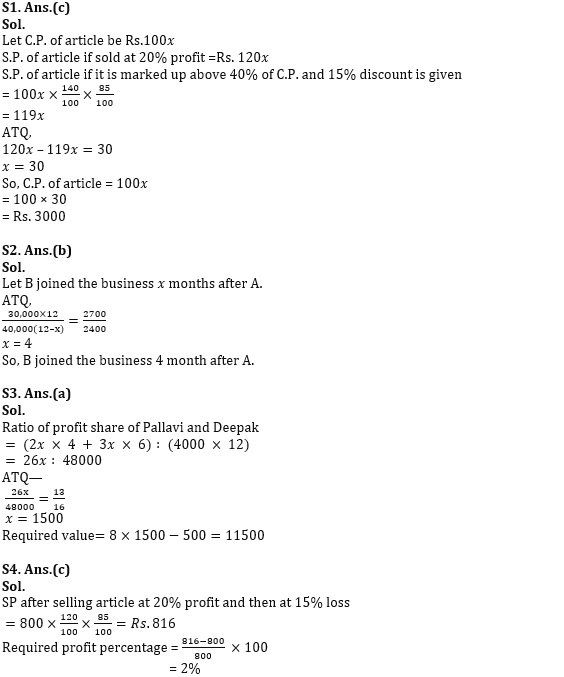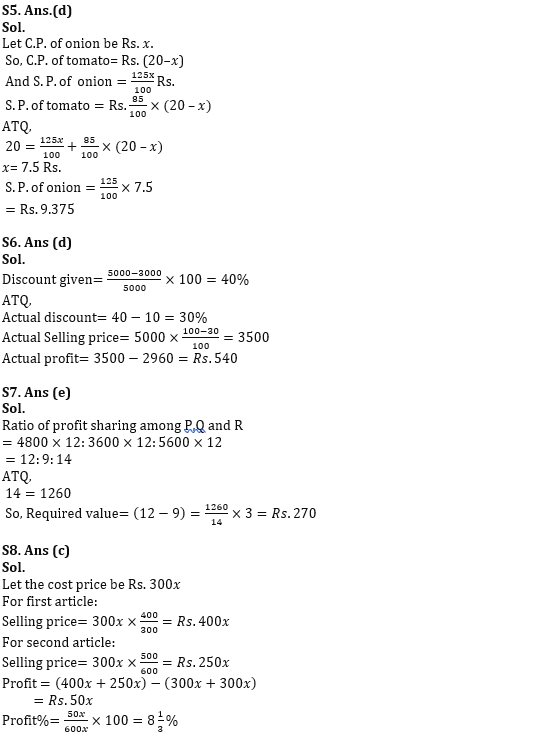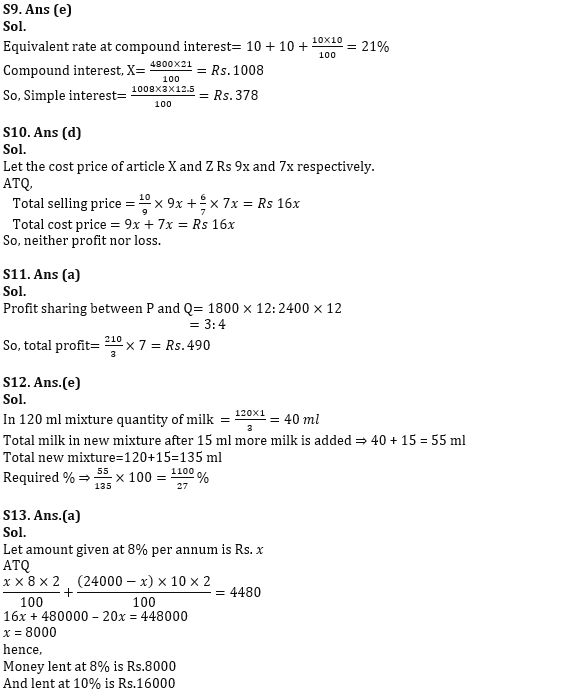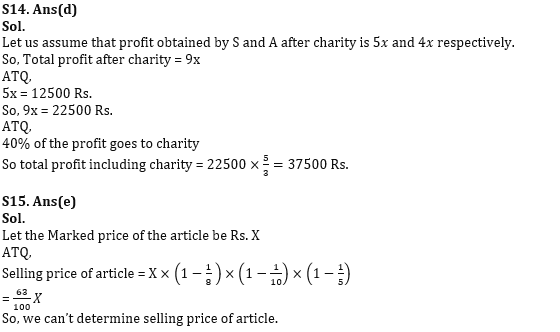#### Congratulations!Incorrect details? Fill the form again here

•Quantitative Aptitude Quiz For IBPS RRB ...
•Quantitative Aptitude Quiz For IBPS RRB ...
•Profit and Loss: Formulas, Concepts, Tri...
•Quantitative Aptitude Quiz For SBI Clerk...
•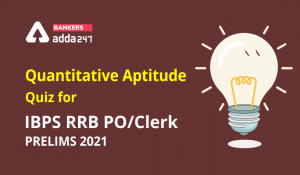Quantitative Aptitude Quiz For IBPS RRB ...
•Quantitative Aptitude Quiz For SBI Clerk...